# NCERT Solutions class 7 Maths Chapter-10 Exercise 10.4

NCERT Solutions Class-7 Maths chapter-10 line and angle Exercise-10.4 is prepared by academic team of pw all the questions of NCERT text book are solved step by step with proper and detail solutions explaining each and every questions . For More and additional questions of CBSE class 7 maths you can go to class 7 maths sections. NCERT class 7 Maths Solutions is the best way to enhanced your mathematics skill. And pw practice worksheet & question bank will help you a lot .

## NCERT Solutions class 7 Maths Chapter-10 line and angle

### Solutions of Chapter line and angle Exercise-10.4

Question 1:
Construct ?ABC, given m∠A = 60°, m∠B = 30° and AB = 5.8 cm.
A rough sketch of the required ?ABC is as follows.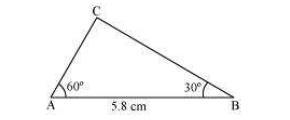The steps of construction are as follows.
(i)Draw a line segment AB of length 5.8 cm.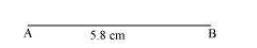(ii)At point A, draw a ray AX making 60º angle with AB.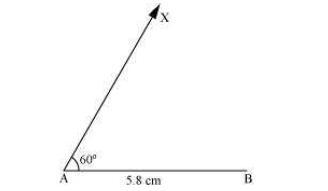(iii) At point B, draw a ray BY, making 30º angle with AB.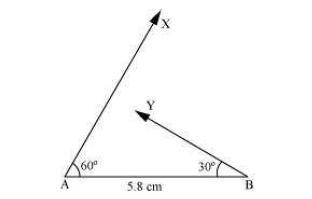(iv) Point C has to lie on both the rays, AX and BY. Therefore, C is the point of intersection of these two rays.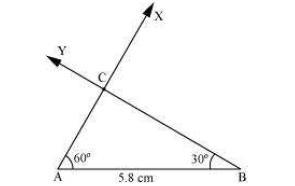This is the required triangle ABC.

Question 2:
Construct ?PQR if PQ = 5 cm, m∠PQR = 105° and m∠QRP = 40°.
(Hint: Recall angle sum property of a triangle).
A rough sketch of the required ?PQR is as follows.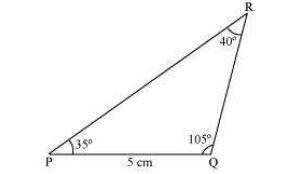In order to construct ?PQR, the measure of ∠RPQ has to be calculated.
According to the angle sum property of triangles,
∠PQR + ∠PRQ + ∠RPQ = 180º
105º + 40º + ∠RPQ = 180º

145º + ∠RPQ = 180º
∠RPQ = 180° − 145° = 35°
The steps of construction are as follows.
(i) Draw a line segment PQ of length 5 cm.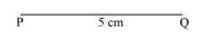(ii) At P, draw a ray PX making an angle of 35º with PQ.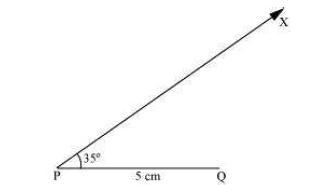(iii) At point Q, draw a ray QY making an angle of 105º with PQ.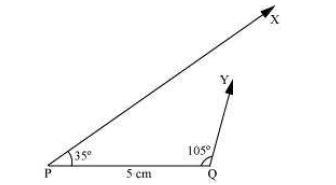(iv)Point R has to lie on both the rays, PX and QY. Therefore, R is the point of intersection of these two rays.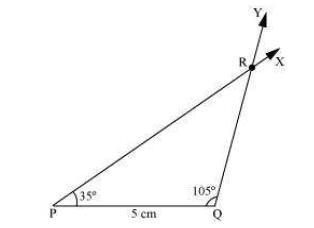This is the required triangle PQR.

Question 3:
Examine whether you can construct DEF such that EF = 7.2 cm, m∠E = 110° and m∠F = 80°. Justify your answer.
Given that,
m∠E = 110° and m∠F = 80°
Therefore,
m∠E + m∠F = 110° + 80° = 190°

However, according to the angle sum property of triangles, we should obtain
m∠E + m∠F + m∠D = 180°
Therefore, the angle sum property is not followed by the given triangle. And thus, we cannot construct ?DEF with the given measurements.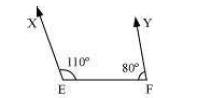Also, it can be observed that point D should lie on both rays, EX and FY, for constructing the required triangle. However, both rays are not intersecting each other. Therefore, the required triangle cannot be formed

NCERT CLASS 7 MATHEMATICS SOLUTIONS